How do you evaluate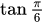?Salma

Solution:For any right triangle,
here are 6 trigonometric ratios: sine (sin), cosine (cos), tangent (tan), cosecant (cosec), secant (sec) and cotangent (cot).

Trigonometric Ratios

Step 1

Consider a triangle ABC, right angled at B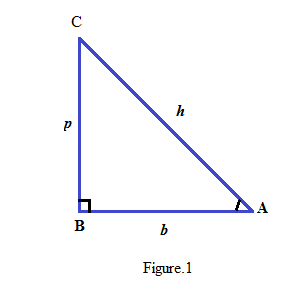Step:2

In the above right triangle ABC, AB is base (b), BC is perpendicular (p) and AC is hypotenuse (h).

Sin is defined as the ratio of perpendicular to hypotenuse, cos is defined as the ratio of base to hypotenuse and tan is defined as the ratio of perpendicular to base. There exists three more ratios which are reciprocal of sin, cos and tan.

For angle A, these ratios can be listed as shown below.tan A is the ratio of sin A and cos A, which can be written as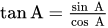.

Cosec A is reciprocal of sin A sec A is reciprocal of cos A.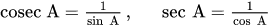Trigonometric ratios of some basic angles as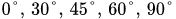are defined.

For some other angles trigonometric ratios can be derived from these ratios.

How to memorize all the trigonometric ratios?

Step:3 There is a table- trick to memorize all these ratios for the angles written above.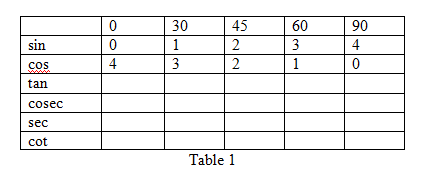In the row of “sin” put the numbers from 0 to 4 in increasing order and then in the row of “cos” put all the numbers from 4 to 0 in decreasing order.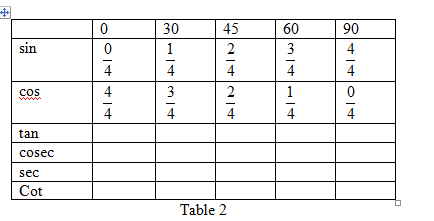Divide all the numbers by 4.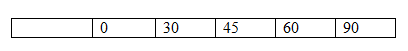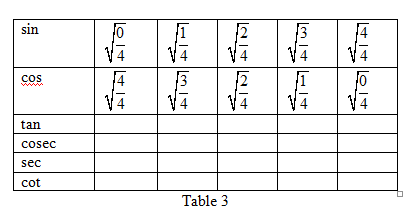Write all the values in square root.

All the values of table can be simplified and written. The value of tan is ratio of sin and cos. Cosec is reciprocal of sin, sec is reciprocal of cos and cot is reciprocal of tan.

The completed table is made hereunder.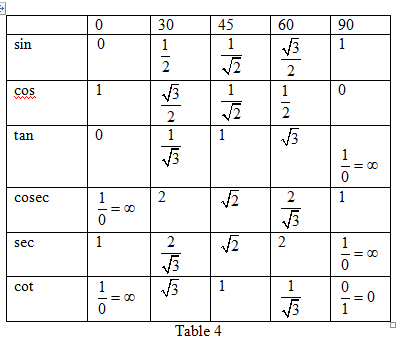To measure the angles two units are used namely radian and degree.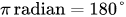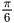radian can be converted into degree using the above conversion.Now to find the value of, evaluate the value of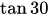.

From Table 4 it can be seen clearly that the value ofis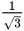.

Explanation of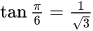:

Consider a right triangle with an angle of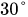.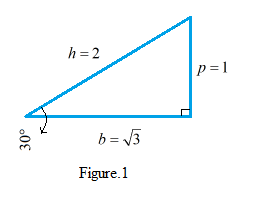Now tan is defined as the ratio of perpendicular and base.

From Figure.1 value ofcan be calculated as follows: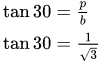Answer. The value ofthat isisSimilar Problems:

Problem 1. What is the value of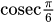?

Solution:

Step:1 To measure the angles two units are used namely radian and degree.radian can be converted into degree using the above conversion.Step:2 Now to find the value of, evaluate the value of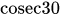.

From Table 4 it can be seen clearly that the value ofis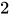.

Answer. The value ofis.

Problem 2. What is the value of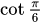?

Solution:

Step:1 To measure the angles two units are used namely radian and degree.radian can be converted into degree using the above conversion.Step:2 Now to find the value of, evaluate the value of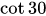.

From the Table 4, it is seen that the value ofis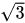.

From the above Figure.1, the value ofis calculated as follows:Answer. The value ofis.

Need homework help now?

tutors online

Related Questions
(t) in °C of the pot of soup at time t (in The initial temperature of a pot of soup is 80°C. The temperature minutes) satisfies the differential equation dT di T-20 10 (a) Solve the differential equation, and find an explicit expression for the function (). By separating variables, integrating and manipulating arbitrary constants, . We are also told that so that and so . ...
Notes
Me

OR

Don't have an account?

Join OneClass

Access over 10 million pages of study
documents for 1.3 million courses.

Join to view

OR

By registering, I agree to the Terms and Privacy Policies
Just a few more details

So we can recommend you notes for your school.